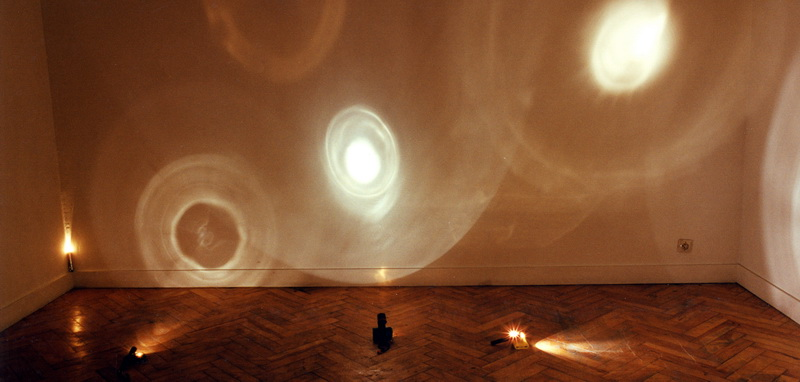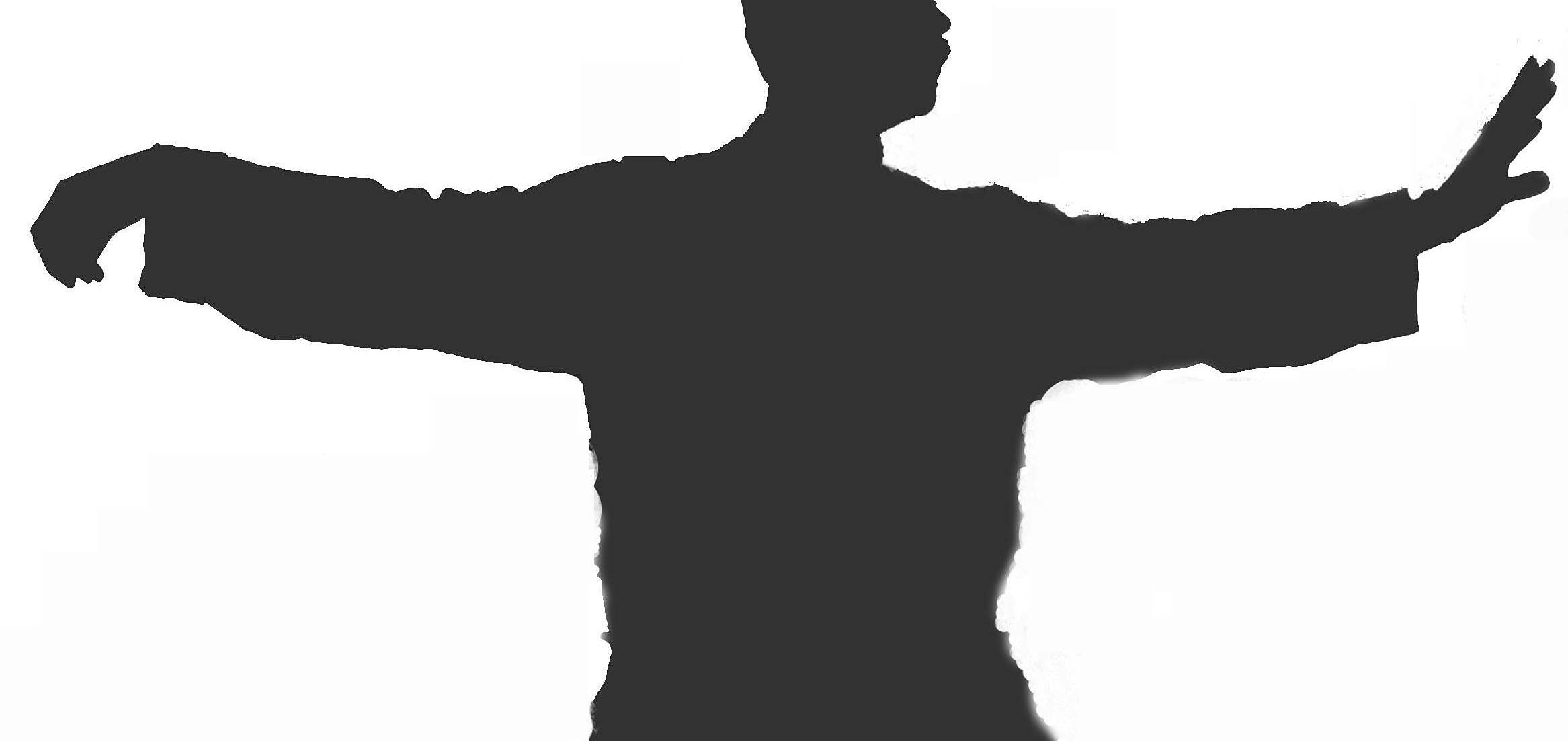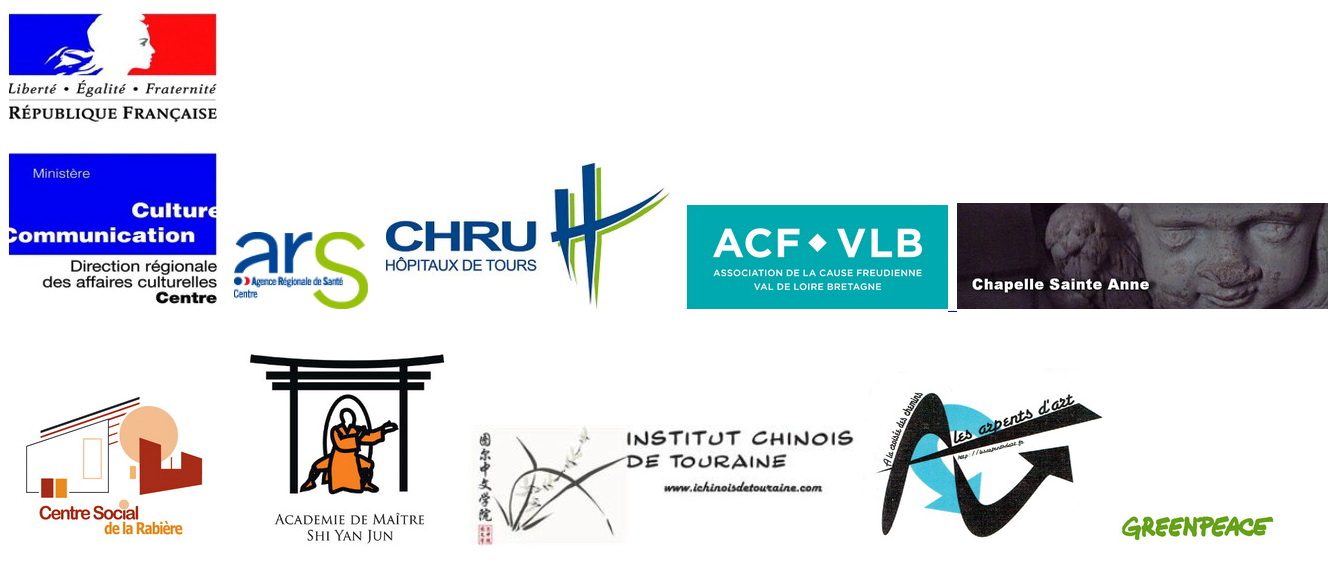Newtopia 4.2

Second chapter of Newtopia 4 …centered on the sensitive body and relative stilness, slow motion…
At the Saint Anne Chapel, in Tours the 2-4 December 2016.

• Exposition-happenings on December 2, 3 et 4  : Expositions, tea ceremony, poetic Tai chi, happenings and somatic practices. Free entry.
Opening hours : 14H-20H on December 2nd and 11H-20H on December 3 & 4.
• Performances on December 2 et 3 at 20H :  vocal, sensorialand choreographic performances. Entry fee : 8€ or free for social minima / Reservation necessary (50 persons only in a session) : net/core/reservations/
• Discussion on December 4 at 14H : discussion psychoanalysis & dance. Free entry. Reservation necessary (limited to 50 persons) : net/core/reservations/

EXPOSITIONS & HAPPENINGS / 2, 3 & 4 of December :

<<<<<<<<<<<<<<<<<<<<<<<<<<<<<<<<<<<<<<<<<<<<<<<<<<<<<<<<<<<<<

Angel Detector (1992)

Interactivity, Sound, Sculpture, Collaborations, Light and Shadow by Jakob Gautel and Jason Karaïndros“Looking into the heart of light, the silence …” – T. S. Eliot

“Un ange passe” – this French expression is taken from the idea that a lull in a conversation occurs when an angel passes by. Silence has become rare in present society, haunted as it is by horror vacui.
The lamp inside the transparent dome only lights up when it is able to detect total silence in its vicinity.
The Angel Detector becomes a sort of lighthouse inviting a passing angel to rest for a moment.
A (silent) dialogue with the angel that has been thus attracted can begin.

Jakob Gautel and Jason Karaïndros

<<<<<<<<<<<<<<<<<<<<<<<<<<<<<<<<<<<<<<<<<<<<<<<<<<<<<<<<<<<<<

CONSTELLATION (1995)

Installation, Light and Shadow, Time by Jason KaraïndrosAbout twenty different flashlights on the floor light parts of the floor, walls and ceiling. The light rays produce auras of differents diametres and intensities, palpitating as the batteries get lower, dimming and extinguishing finally as dying stars. Every day batteries have to be changed, a real waste of energy.

Plus d’info sur l’œuvre et l’artiste : jasonkaraindros.net/constellation

<<<<<<<<<<<<<<<<<<<<<<<<<<<<<<<<<<<<<<<<<<<<<<<<<<<<<<<<<<<<<

Imprint of Our Encounter (1992)

Portraits, Installation, Drawing, Time, Science, Performance, Lines / Variable dimensions.How to draw double portraits, or how to ”photograph” yourself with your friends.

For my personal show at the Beaux-Arts Gallery in Paris in 1992, I tried out for the first time this installation. An armchair where visitors are invited to have a seat, a table with an electrocardiograph which the Cochin Hospital lent me graciously, and an action that takes consecutively place, with the visitors’ participation.
I pre-registered my own electrocardiograph and put the paper back in the machine. I then took the visitor’s electrocardiograph, printing it over mine, the lines running in parallel and sometimes crossing each other. Part of these two meeting lines is a gift for the visitor, the other is put up on the wall, encounter after encounter.
The work’s title could also be ”Affairs of the Heart”.

<<<<<<<<<<<<<<<<<<<<<<<<<<<<<<<<<<<<<<<<<<<<<<<<<<<<<<<<<<<<<

SOMA SENSIBILIS (2016)

Somatic education matters

Free sessions after registration : net/core/reservations/

Promoting introspection and slow action : Body Mind Centering (Marie Foulatier,Cécilia Ribault), Feldenkrais (Marie-Claude Farel, Elisabeth Mercier Rafin), Pédagogie Perceptive (Christine Merlin, Laetitia Bourgoin).

<<<<<<<<<<<<<<<<<<<<<<<<<<<<<<<<<<<<<<<<<<<<<<<<<<<<<<<<<<<<<

L’HOMME MASQUE (2016)

Martial arts performances and slow dances during  the expositionTai Chi (Chen style), slow dances & masks by France Shaolin and Omnivion members.

www.franceshaolin.fr

<<<<<<<<<<<<<<<<<<<<<<<<<<<<<<<<<<<<<<<<<<<<<<<<<<<<<<<<<<<<<

SILENCE IS NOT THE ABSENCE OF SOUND

Sensitive listening: listen to recordings of the last places of the globe without sound pollution.

[Gordon Hempton] believes that only about 50 locations are free from the noise of human activity. “Before,” he said, “we could sometimes have several consecutive hours of silence. Now it’s just about twenty minutes. (Le Monde, 2016) / quietplanet.com/
<<<<<<<<<<<<<<<<<<<<<<<<<<<<<<<<<<<<<<<<<<<<<<<<<<<<<<<<<<<<<

TEA TIME (2016)

Chinese tea ceremony during the exposition

Meditative Infusions, by Xueqin Bonneau from the Chinese Institute of Tours. A silent reciprocity around a re-contextualized traditional practice. Visitors of the exhibition may possibly enjoy a tea with the hosts.

<<<<<<<<<<<<<<<<<<<<<<<<<<<<<<<<<<<<<<<<<<<<<<<<<<<<<<<<<<<<<

PERFORMANCES / December 2 & 3 at 20H :

Entry fee 8€ (free for social minima) / Reservation necessary (50 persons only in a session) : net/core/reservations/

SILENCE OF THE GOATS (2016)

Postural and vocal performance

Dimitri Tsiapkinis in a collaboration with Roland Lebret and Claire Guillaume.

<<<<<<<<<<<<<<<<<<<<<<<<<<<<<<<<<<<<<<<<<<<<<<<<<<<<<<<<<<<<<

SIRENES (2016)

Postural and vocal performance

A duet withMagali Dijoux and Carl Loiseau. Sensitive singing and dance.

<<<<<<<<<<<<<<<<<<<<<<<<<<<<<<<<<<<<<<<<<<<<<<<<<<<<<<<<<<<<<

Flowering throats (2016)

Gestural and respiratory performances

Magali Dijoux, Claire Guillaume, Bruno Morin and Rosa Pires de Matos propose sensorial studies with the poem « Mbo » by Gérard Haller.

<<<<<<<<<<<<<<<<<<<<<<<<<<<<<<<<<<<<<<<<<<<<<<<<<<<<<<<<<<<<<

Untitled (2016)

Dance and cello performance

An improvisation on slow action and silence by Dimitri Tsiapkinis and Soïzic Lebrat.

www.soiziclebrat.eu

<<<<<<<<<<<<<<<<<<<<<<<<<<<<<<<<<<<<<<<<<<<<<<<<<<<<<<<<<<<<<

Looking at dance  (2016)

Psychoanalysis and dance

A conversation between dancer-choreographer Dimitri Tsiapkinis and psychoanalyst Ariane Oger, moderated by psychologist Isabelle Buillit.

Reservation necessary (limited to 50 persons) : net/core/reservations/

<<<<<<<<<<<<<<<<<<<<<<<<<<<<<<<<<<<<<<<<<<<<<<<<<<<<<<<<<<<<<

OUR PARTNERS :